# 6 Variable K-Map in Digital Electronics

Read this article to learn how you can use the K-Map (Karnaugh Map) to reduce a Boolean function in six variables. Let's start with a brief introduction to Karnaugh Map (K-Map).

## Karnaugh Map (K-Map)

The Karnaugh Map or K-Map is a graphical method of reducing a Boolean function to its minimal form. The K-Map can be defined as a chart or a graph that is composed of an arrangement of adjacent squares or cells, where each cell represents a particular combination of variables of the Boolean expression either in sum or product form. A typical 2 Variable K-Map is represented in Figure-1.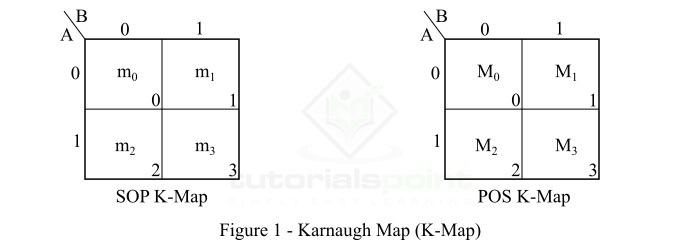In actual practice, we generally use a K-map upto 6 variables. However, K-map can be used for any number of variables, but for variables 5 or more, it becomes tedious.

Now, let us discuss the six variable K-map and its application to reduce a Boolean function to the minimal form.

## Six Variable K-Map

A six variable K-Map is used to reduce a Boolean expression of six variables (say A, B, C, D, E, F). It has 64 (26) adjacent cells or squares. Where, each cell represents a combination of variables of the function.

For a 6-variable Boolean function in SOP form, the possible combinations of the input variables are as follows −

$$\bar{A}\bar{B}\bar{C}\bar{D}\bar{E}\bar{F},\bar{A}\bar{B}\bar{C}\bar{D}\bar{E}F,\bar{A}\bar{B}\bar{C}\bar{D}E\bar{F},\cdot \cdot \cdot ABCDEF$$

The minterm designations of these combinations of variables are m0, m1, m2 … m63 respectively.

Similarly, for a 6-variables Boolean function in POS form, the possible combinations of input variables are as follows −

$$\left ( A\,+\,B\,+\,C\,+\,D\,+\,E\,+\,F \right ),\left ( A\,+\,B\,+\,C\,+\,D\,+\,E+\bar{F} \right ),\left ( A\,+\,B\,+\,C\,+\,D+\bar{E}+F \right ),\cdot \cdot \cdot \cdot \left ( \bar{A}+\bar{B}+\bar{C}+\bar{D}+\bar{E}+\bar{F} \right )$$

The maxterm designations of these combinations of variables are M0, M1, M2, …M63 respectively.

As already mentioned, the six variable K-map has 64 cells which are divided into 4-blocks of 16 squares each. Each cell on the K-map represents a minterm or a maxterm. In the case of 6 variable K-map, the values of variables A and B remain the same for all minterms (or maxterms) in each block of 16 squares.

The 64 cells of a six variable K-map are divided into 4 blocks as follows −

• Block 1 − This is the top left block. This block represents minterms from m0 to m15, (or maxterms from M0 to M15). In this block, the variable A is a 0 and the variable B is also a 0.

• Block 2 − This is the top right block. This block represents minterms from m16 to m31, (or maxterms from M16 to M31). In this block, the variable A is a 0 and the variable B is a 1.

• Block 3 − This is the bottom left block. This block represents minterms from m32 to m47, (or maxterms from M32 to M47). In this block, the variable A is a 1 and the variable B is a 0.

• Block 4 − This is the bottom right block. This block represents minterms from m48 to m63, (or maxterms from M48 to M63). In this block, the variable A is a 1 and the variable B is also a 1.

While simplification, the 6-variable K-map may contain 2-squares, 4-squares, 8-squares, 16- squares, 32-squares, or a 64-square by involving all the four blocks of the map.

In a six variable K-Map, when a block is superimposed on the top of another block, which is either above or below or beside of the first block, and the squares coincide with one another. Then, the squares are considered adjacent in the two blocks. It is also important to note that the diagonal elements such as m10 and m58, m15 and m63, m18 and m34, m29 and m45 are not adjacent to each other.

A six variable SOP K-map is represented in Figure-2.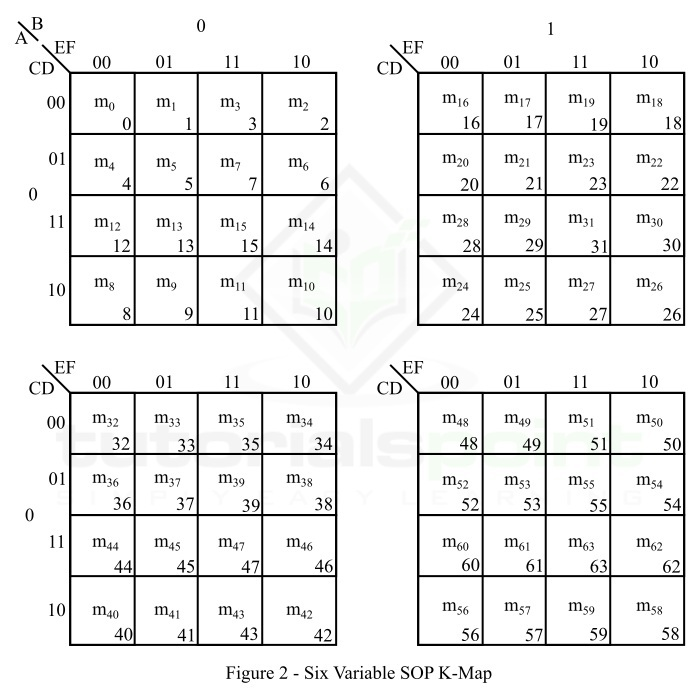A six variable POS K-map is represented in Figure-3.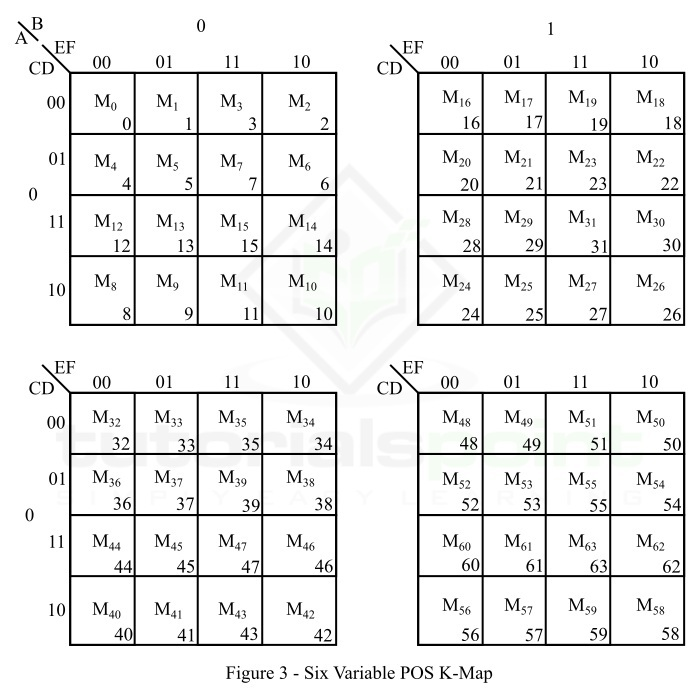Now, let us understand the utilization of the six variable K-map for minimization of a six variable Boolean function with the help of solved numerical examples.

## Example 1

Minimize the following 6 variable Boolean function using K-map.

$$f=\sum m( 1, 3, 4, 5, 6, 9, 11, 12, 14, 15, 17, 19, 20, 21, 22, 23,25, 27, 28, 30, 33, 35, 36, 38, 41, 43, 44, 46, 49, 51, 52, 54, 57, 59, 60, 62)$$

### Solution

The 6-variable SOP K-map representation of the given Boolean function is shown in Figure-4.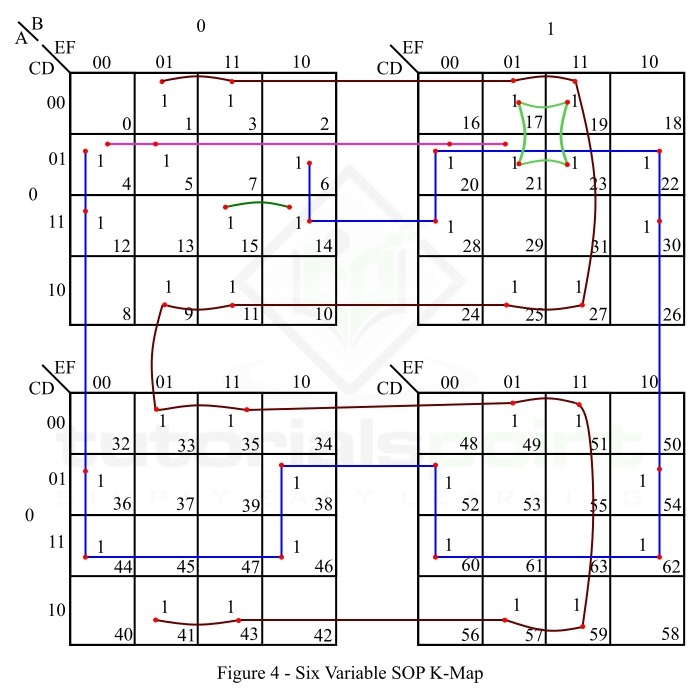The reduction of this function is done as per the following steps −

• There are no isolated 1s in the K-map.

• The minterm m1 forms a 16-square with minterms m3, m9, m11, m17, m19, m25, m27, m33, m35, m41, m43, m49, m51, m57, and m59. Make it and read it as $\bar{D}F$.

• The minterm m4 forms a 16-square with minterms m6, m12, m14, m20, m22, m28, m30, m36, m38, m44, m46, m52, m54, m60, and m62. Make it and read it as $\bar{F}D$.

• The minterm m5 can form a 4-square with minterms m4, m20, and m21, or with m1, m17, and m21. We will make it with minterms m4, m20, and m21, and read it as $\bar{A}\bar{C}D\bar{E}$.

• The minterm m21 forms a 4-square with minterms m17, m19, and m23. Make it and read it as $\bar{A}B\bar{C}F$.

• The minterm m15 can make a 2-square with the minterm m11 or m14. We will make it with m14, and read it as $\bar{A}\bar{B}CDE$.

• Write all the product terms in SOP form.

Therefore, the minimal SOP expression is,

$$f\left ( A,B,C,D,E,F \right )=\bar{D}F+D\bar{F}+\bar{A}\bar{C}D\bar{E}+\bar{A}B\bar{C}F+\bar{A}\bar{B}CDE$$

## Example 2

Minimize the following six variable Boolean function using K-map.

$$f=\Pi M(0, 2, 7, 8, 10, 13, 16, 18, 24, 26, 29, 31,32, 34, 37, 39, 40, 42, 45, 47, 48, 50, 53, 55, 56, 58, 61, 63)$$

### Solution

The 6-variable POS K-map representation of the given Boolean function is shown in Figure-5.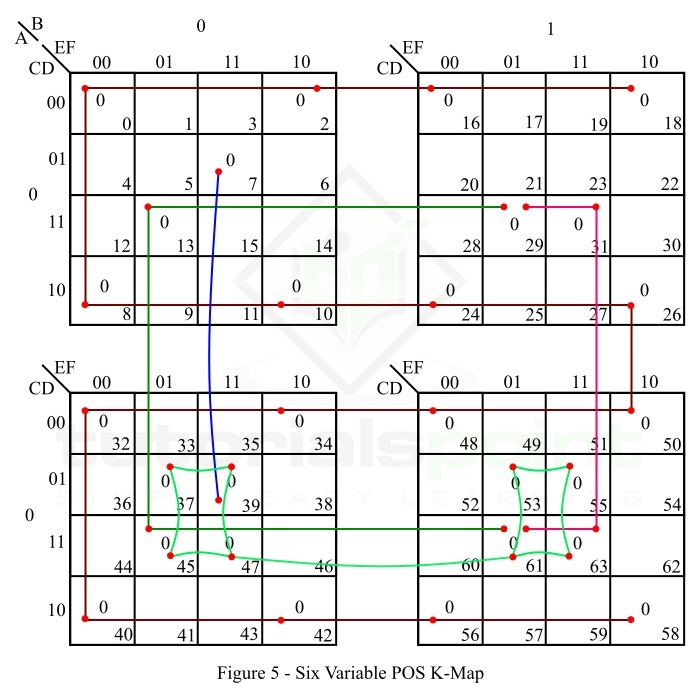The reduction of this function is done as per the following steps −

• There are no isolated 0s in the K-map.

• The maxterm M0 forms a 16-square with maxterms M2, M8, M10, M16, M18, M24, M26, M32, M34, M40, M42, M48, M50, M56, M58. Make it and read it as $\left ( D+F \right )$.

• The maxterm M37 forms an 8-square with maxterms M39, M45, M47, M53, M55, M61, and M63. Make it and read it as $\left ( \bar{A}+\bar{D}+\bar{F} \right )$.

• The maxterm M13 makes a 4-square with maxterms M29, M45, and M61. Make it and read it as $\left( \bar{C}+\bar{D}+E+\bar{F} \right )$.

• The maxterm M31 makes a 4-square with M29, M61, and M63. Make it and read it as $\left( \bar{B}+\bar{C}+\bar{D}+\bar{F} \right )$.

• The maxterm M7 forms a 2-square with the maxterm M39. Make it and read it as $\left( B+C+E+\bar{D}+\bar{E}+\bar{F} \right )$.

• Write all the sum terms in POS form.

Thus, the minimal POS expression for the given Boolean function is,

$$f\left ( A,B,C,D,E,F \right )=\left ( D+F \right )\left ( \bar{A}+\bar{D}+\bar{F} \right )\left( \bar{C}+\bar{D}+E+\bar{F} \right )\left( \bar{B}+\bar{C}+\bar{D}+\bar{F} \right )\left( B+C+E+\bar{D}+\bar{E}+\bar{F} \right )$$

This is all about six variable K-map and its application in minimization of Boolean functions. Try solving the following tutorial problems to excel in the concept of six variable K-map and its application to reduce Boolean expressions.

Q. 1 − Minimize the following six variable Boolean function in SOP form using K-map.

$$f\left ( A,B,C,D,E,F \right )=\sum m(1, 3, 5, 7, 9, 10, 11, 12, 14, 16, 17, 19, 20, 22, 24, 25, 26, 31, 32,34, 36, 37, 39, 40, 42, 45, 49, 50, 53, 54, 57, 60, 61, 63)$$

Q. 2 − Minimize the following six variable Boolean function in POS form using K-map.

$$f\left ( A,B,C,D,E,F \right )=\Pi M( 0, 1, 3, 4, 5, 6, 8, 9, 10, 12, 14, 15, 17, 20, 21, 22, 25, 26, 27,29, 30, 32, 33, 35, 36, 37, 39, 40, 41, 42, 45, 47, 48, 50, 52, 53, 55, 56, 58, 59, 60, 62 )$$

Updated on: 19-Apr-2023

1K+ Views